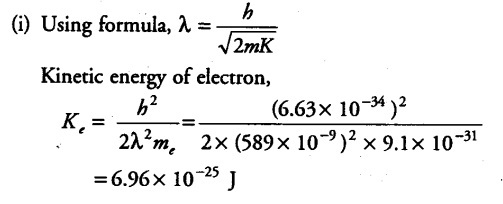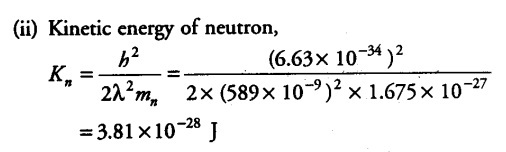# The wavelength of light from the spectral emission line

The wavelength of light from the spectral emission line of sodium is 589 nm. Find the kinetic energy at which
(i) an electron and
(ii) a neutron would have the same de-Broglie wavelength?

The wavelength of light from the spectral emission line = 589 nm = 589 x {{10}^{-9}} m
Mass of electron, { M }_{ e } = 9.1 x {{10}^{-31}} kg
Mass of neutron, { M }_{ n } = 1.675 x {{10}^{-27}} kg
Planck’s constant , h =6.63 x {{10}^{-34}} J/Kg